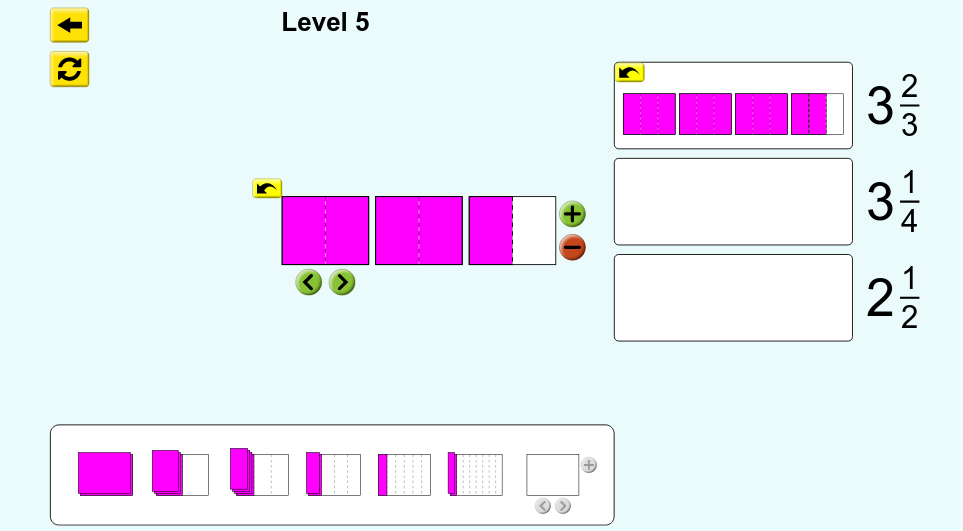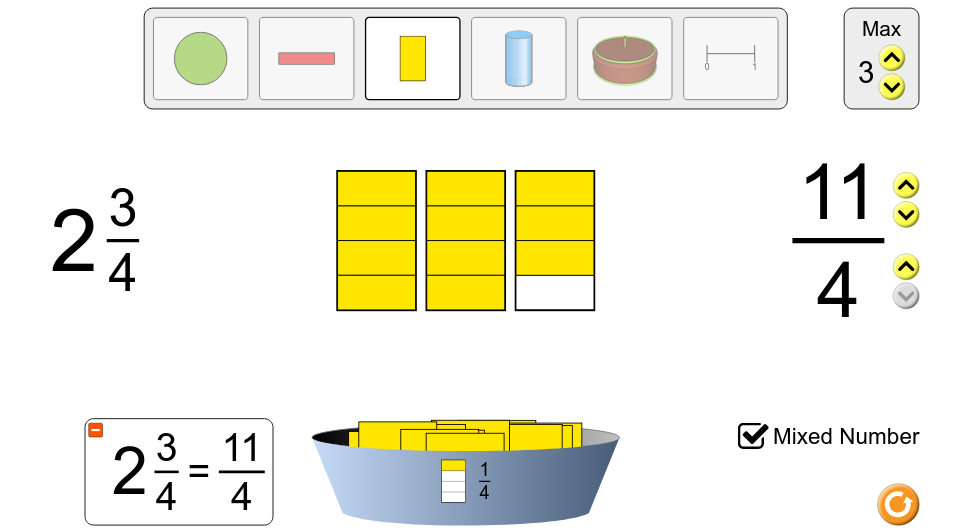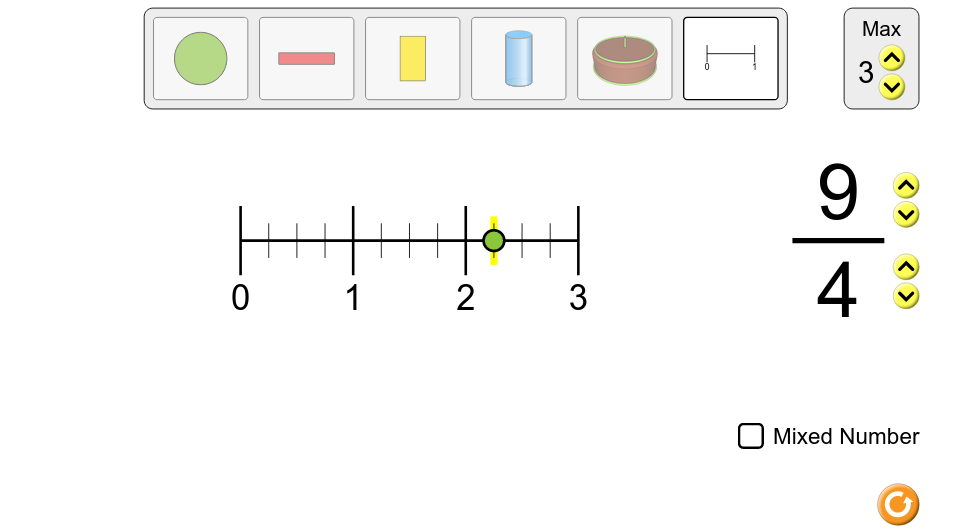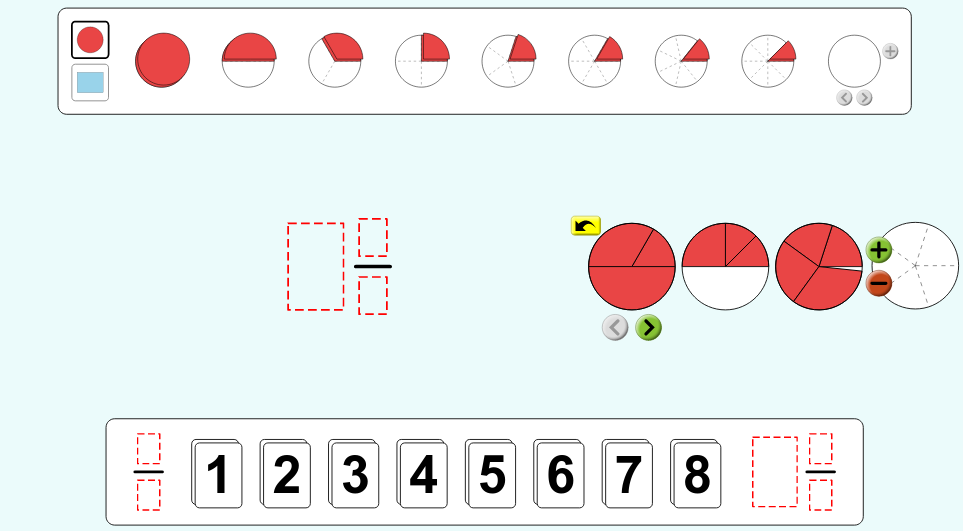MM Practice
×
Multiplication
Division
Place Value
Fractions & Decimals
Measurement
Statistics & Probability
Pre-Algebra
Money
Kindergarten
Geometry

# Mixed Numbers with Visual Models interactive activities and game (grades 3-5)

These activities allow you to explore mixed numbers and improper fractions with the help of visual models and the number line. You can also play a game where you make mixed numbers from visual models.

There are three sections:

1. In the first section (Intro), you will first of all build improper fractions using different visual models or a number line. You can choose the numerator and the denominator of the fraction, to see how it is illustrated with a visual model or on a number line. Then you can choose to show it as a mixed number.

This is a great place for students to investigate how the numerator and the denominator of a fraction actually work and what happens if you increase/decrease one or the other.

Teachers can use this to illustrate fractions and mixed numbers on a number line.

2. The second section is a game where you are asked to build different mixed numbers using the given pie slices.
3. Lastly, in the "Lab" section you can play with different size pieces (either a pie model or a rectangular model). You can put, say, ⅙ and ⅓ into the same circle and see what you get. Or, you might put two fifths into the circle, then a fourth, then a third, and notice it doesn't quite make one full "pie" — there is a little empty slice left.

Teachers can use this section to illustrate equivalent fractions.

These activities best suit third and fourth grades.

Credit: This activity is created by PhET

Screenshots from the game and activities:Allow my comment to be posted on this site